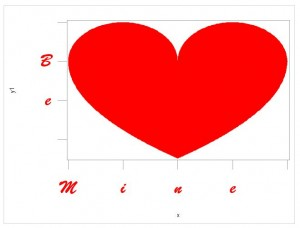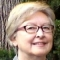SAS Code for your Valentine - just in case you forgot to get flowers, chocolate or other tokens of affection for the big day yesterday.

proc format;
value x  .5='e' 0='n' -.5='i' -1='M' other=' ';
value y -1='e' 0='B' other=' ';
run;

data h;
pi = constant("PI");
do r =  0 to pi by .02;
x = cos(r)*.5+.5;y1 = sin(r);y0 = 2.5*sin(r/2+pi);output;
x=-x;output;
end;
run;
proc sort ;by x;run;

proc sgplot data=h;
band x=x upper=y1 lower=y0/fillattrs=(color=CXFF0000);
format x x. y1 y0 y.;
xaxis valueattrs=(color=CXFF0000 weight=bold size=30 family="Brush Script MT");
yaxis valueattrs=(color=CXFF0000 weight=bold size=30 family="Brush Script MT");
run;

Here's what you will get:Rick Wicklin uses SAS/IML Studio to provide a different parameterization.  If my Valentine doesn't get you out of the dog house, read his post - A parametric view of love.

SharePrincipal Software Developer

Clarke Thacher has been using SAS since he first loaded a deck of cards to analyze health care data in 1975. He has worked in SAS R&D since 1985 and still enjoys using SAS to produce insight out of a cloud of data

1.Hi
I am getting an error message when I try and run the code
•